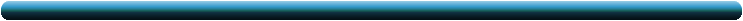CMSIM.netHome | Christos H Skiadas | Nonlinear Regression with Excel | First Exit Time Models-Applications | Gompertz Models | Longevity | Life Expectancy Estimates | Life Expectancy at Birth in Netherlands | Health State Function | Healthy Life Expectancy | Healthy Life Expectancy in Scotland | Human Development Stages | Health State Life Table

Nonlinear Regression-First Exit Time ModelsA new nonlinear regression package in Excel for simple mortality models is available here: click here to download Simple Mortality Models Nonlinear Regression  The best fit model is a combination of a model derived from the first exit time theory of a stochastic process with a Gompertzian correction for the ages from 15 to 30 years.  For the best fit model go to the Health State Model webpage Please download the latest version of the Regression model in ExcelPeople trying nonlinear regression to fit a nonlinear function to data usually face the problem of finding special programs and "pacquages" and invest time to learn and apply.
Why not to try a nonlinear regression in Excel?

In this page we introduce a program of Nonlinear Regression Analysis in Excel for the estimation of parameters of models fitting to the age distribution of deaths for specific populations.
Many applications refer to the data provided by the National Statistics or Health Statistics or the Bureau of the Census of various countries or by specialized institutes (The data used in our applications are mainly from the Human Mortality Database, www.mortality.org)
The annual data per age of death, usually from 0 to 110 are divided by their total number so that there appear a probability distribution which makes easy the comparisons between different time periods or various countries or population groups.

We also give two models based on the First Exit Time Theory of a stochastic process which in this case is the Human Health State.
The first model avoids the infant mortality while provides good fitting for the remaining part of the data sets: http://www.cmsim.net/sitebuildercontent/sitebuilderfiles/skiadasamodelingapproach.pdf
whereas the second model (IM) takes into account the infant mortality (see Skiadas, C. and Skiadas, C. H., Development, Simulation and Application of First Exit Time Densities to Life Table Data, Communications in Statistics - Theory and Methods, Volume 39, Issue 3 January 2010, pages 444 - 451. This paper can be downloaded from: http://www.informaworld.com/smpp/content~db=all~content=a918382403~frm=titlelink).
We also provide a Nonlinear Regression Analysis Program in Excel for the Weibull Model for applications with Life Table Data.

This method for nonlinear regression uses the method proposed in Skiadas 1986:

The method linearizes a nonlinear function by using the first order terms of a Taylor series expansion in respect to the parameters of the model. Then the parameters are estimated by a minimization of the sum of squared errors following an iterative procedure which is terminated when the errors of the estimates are sufficiently small. In the program written in Excel the iterations are 40 enough for the models and the data sets applied. The estimated values of the parameters and the errors of the estimates are presented along with the standard error and the Sum of Squared Errors SSE and the R2.
As the nonlinear regression analysis method requires a first "guess" of the original parameters to "start" the iterative procedure a graph is introduced. You can see and decide for the better starting point.
Another graph provides the final regression curve and the raw data line. Other graphs provide information for the first and second derivatives and characteristic points as maxima or minima and inflection points, the mortality and the survival curves.

The original program was written in one of the first then programming languages called BASIC in 1982 and then passed to GW BASIC and later in QBASIC.
Recently (July 2010) the iterative program was written in EXCEL 2003 to be possible to anyone to use the program easily and without the need of special tools.
The nonlinear program is now written for the users of mortality data of a population obtained form the Bureau of the Census of various countries or from data bases in specialised institutes as is the Human Mortality Database (www.mortality.org).
The users can have immediately the results and the appropriate graphs for the specific case and many other statistics.

Please refer this web site (http://www.cmsim.net) in your applications of the nonlinear regression analysis programs and the related publications above and send me a copy of your work at: skiadas@asmda.netThe first exit time model program in excel, C. Skiadas

Download the Excel Program for Nonlinear Regression of the First Exit Time Model for Life Table Data (ZIP format)Fit curves of the first exit time model program in excel, C. Skiadas

Download the Excel Program for Nonlinear Regression of the First Exit Time Model for Life Table DataThe first exit time model IM program in excel

Download the Excel Program for Nonlinear Regression of First Exit Time Model-IM (ZIP format)Fit curves of the first exit time model IM program in excelThe Weibull modelWeibull fit curves